Tapping into mathematics

Start this free course now. Just create an account and sign in. Enrol and complete the course for a free statement of participation or digital badge if available.

Free course

# 5.2 Value Added Tax (VAT)

There is some information about Value Added Tax (VAT) on the receipt. VAT is charged on many goods purchased in the UK. At the time of the purchase on this receipt, the VAT rate was 17.5%. This means that on every £100 net cost, £17.50 would be charged in VAT, bringing the total to £117.50. In other words:

Net cost + VAT = Gross cost

£100.00 + £17.50 = £117.50

So the shop would charge £100, the tax office £17.50 and the customer would pay £117.50.

Because you may want to use the VAT rate frequently, it may be convenient to store it in one of your calculator's memories. The TI-84 has lots of memories, represented by the letters of the alphabet, which are the third functions above many of the keys. If you have a different calculator, find out how to store values in its memories. If you have one labelled V, then it may be useful to use it to store the VAT rate.

On the TI-84, there is a V above the 6 key; this indicates that V is the third function of this key. To access it you press and release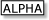followed by this key. To store the value in V, you will need the store key. On the TI-84, this is the key above ON. It is labelled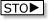.

Remember that the VAT rate is given as a percentage and it is really a fraction made up of this number divided by 100. So the VAT rate is 17.5/100 (i.e. 17.5%). To store this in the memory V on the TI-84, press the following keys:

17.5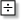100V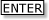If you have set your calculator to display two decimal places then .18 will appear on the display instead of .175. But rest assured that .175 will have been stored in the memory. It will probably remain there even when the calculator is switched off.

Now you can do lots of VAT calculations, using the memory. For example to calculate the VAT on a payment of £72, press:

72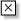VYour calculator should display 12.60 (assuming that you have it set to display two decimal places), so the VAT is £12.60.

MU120_4MCAL